# 金刚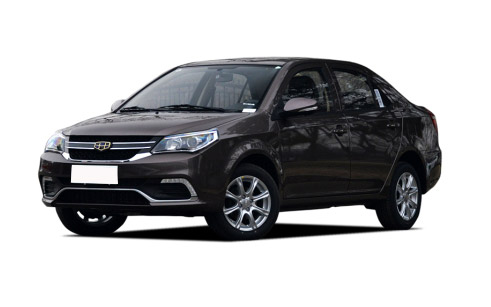•外观
•中控
•座椅
•细节
 本地参考价： 4.79 万元起 厂商指导价：4.79-6.59 万
 车市行情　｜　金刚 降万
 最新年款： 2016款 级　别：小型车 结　构：4门5座三厢车 车身颜色： 红色 油　耗：6.8L/100km 排　量：1.5L 内饰配色： 红黑 保　修：3年或6万公里 变速箱：4挡自动|5挡手动

#### 吉利金刚CROSS正式上市 5.29-6.59万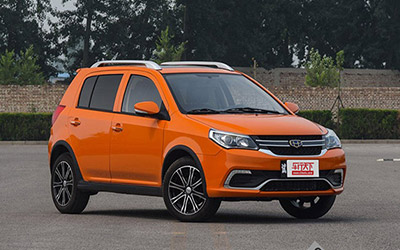#### 变化幅度大 实拍2014款吉利金刚三厢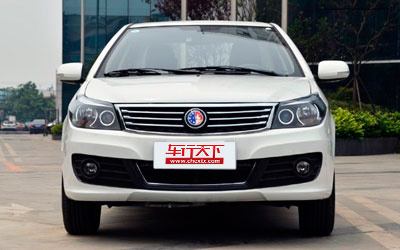#### 实拍金刚二代1.5基本型 自主品牌进化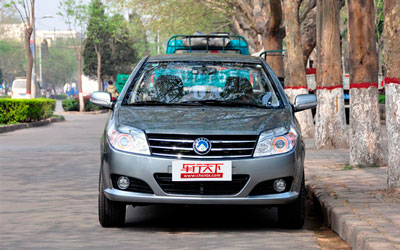1.5L 自然吸气 102马力 国V 官方指导价 本地参考价 车型信息 2016款 1.5L 手动进取型 国V 5挡手动 前置前驱 4.79万暂无 参数　图片　＋对比 2016款 1.5L 手动精英型 国V 5挡手动 前置前驱 4.99万暂无 参数　图片　＋对比 2016款 1.5L 自动尊贵型 国V 4挡自动 前置前驱 6.59万暂无 参数　图片　＋对比 2016款 CROSS 1.5L 手动悦享版 5挡手动 前置前驱 5.29万暂无 参数　图片　＋对比 2016款 CROSS 1.5L 手动智享版 5挡手动 前置前驱 5.59万暂无 参数　图片　＋对比 2016款 CROSS 1.5L 自动尊享版 4挡自动 前置前驱 6.59万暂无 参数　图片　＋对比
 官方指导价 本地参考价 车型信息 2016款 1.5L 手动精英型 国IV 4.79万暂无 参数　图片　＋对比 2016款 1.5L 手动进取型 国IV 4.59万暂无 参数　图片　＋对比

### 绕车实拍 外观中控座椅细节全部### 金刚 新闻资讯#### 吉利金刚CROSS正式上市 5.29-6.59万#### 变化幅度大 实拍2014款吉利金刚三厢#### 实拍金刚二代1.5基本型 自主品牌进化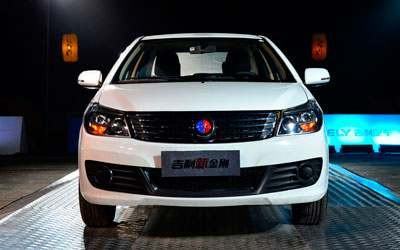#### 测试吉利金刚 刹车性能大幅度提高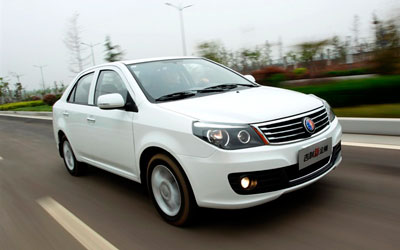#### 吉利新款金刚系列上市 售5.38-6.68万元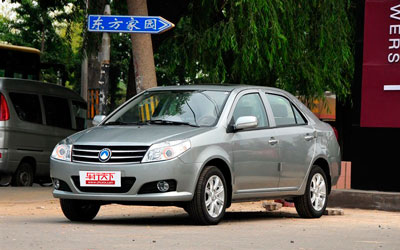#### 英伦金刚/金鹰将被召回 涉及55018辆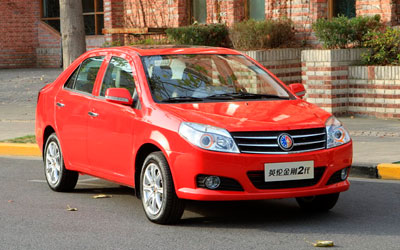#### 金刚/金鹰搭载1.5VVT发动机 动力全新升级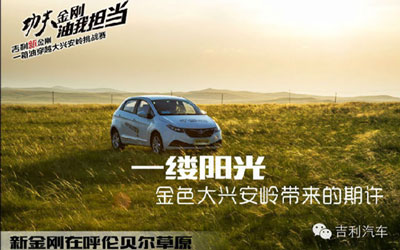#### 吉利新金刚越大兴安岭挑战赛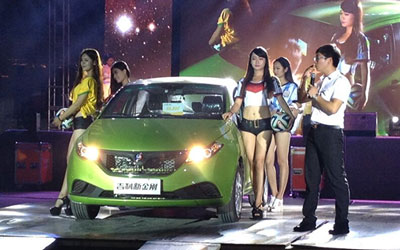#### 金刚邀您共度世界杯冠军之夜

2014年7月13日，啤酒节晚上正是熬夜看世界杯总决赛的最好时光，这时吉利云阳海携带吉利金刚陪您共度2014年世界杯总决赛的激情夜晚。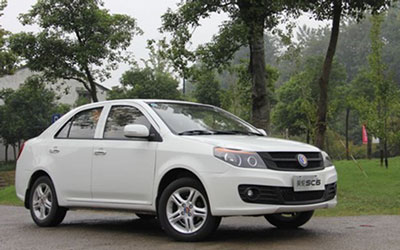#### 家庭代步车型强烈推荐金刚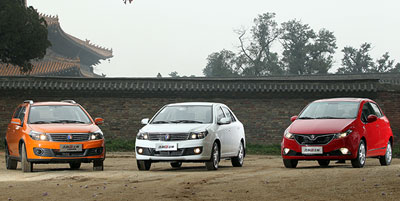#### 吉利新金刚大兴安岭挑战赛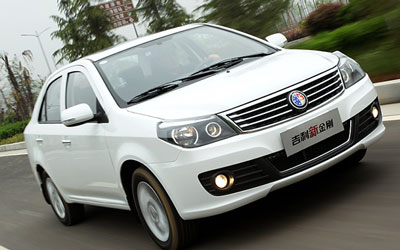#### 吉利新金刚最高优惠8000元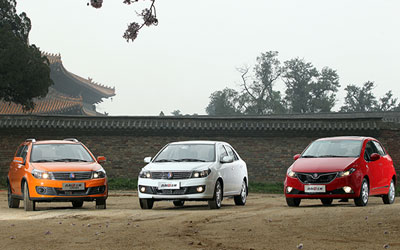#### "合资车型"吉利新金刚叫板桑塔纳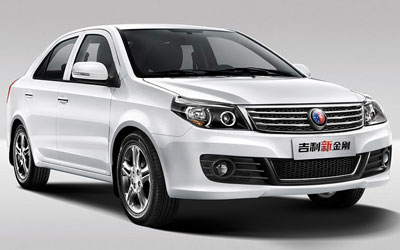#### 云阳海吉利新金刚全新升级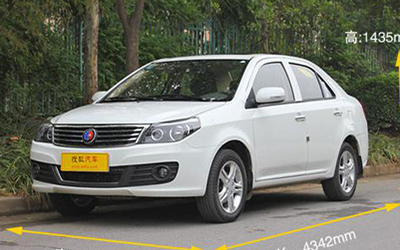#### 5.1河南云阳海 14款金刚独家特售

2014年4月13日在嵩山少林寺正式上市的全新金刚，采用全新最新家族式造型设计，搭载全新1.5L自然吸气发动机，现河南云阳海店内有现车销售。

### 猜你喜欢

﻿
• 快速找车
• 选择品牌
• 选择品牌
• A  奥迪
• A  阿斯顿·马丁
• A  阿尔法·罗密欧
• B  宝沃
• B  布加迪
• B  巴博斯
• B  保时捷
• B  宾利
• B  奔驰
• B  宝马
• B  本田
• B  别克
• B  标致
• B  比亚迪
• B  宝骏
• B  北汽制造
• B  北汽新能源
• B  北汽幻速
• B  北汽威旺
• B  北京汽车
• B  奔腾
• B  北汽绅宝
• B  北汽昌河
• C  长安欧尚
• C  长安
• C  长安凯程
• C  长城
• D  大众
• D  道奇
• D  DS
• D  东南
• D  东风风神
• D  东风风行
• D  东风小康
• D  东风风度
• D  东风
• F  福特
• F  丰田
• F  菲亚特
• F  法拉利
• F  福田
• F  福迪
• F  福汽启腾
• G  观致
• G  广汽传祺
• G  广汽吉奥
• G  GMC
• H  红旗
• H  汉腾汽车
• H  哈弗
• H  哈飞
• H  海格
• H  海马
• H  华颂
• H  黄海
• H  华泰
• H  恒天
• J  捷途
• J  几何汽车
• J  捷达
• J  吉利汽车
• J  捷豹
• J  Jeep
• J  江淮
• J  江铃
• J  金杯
• J  九龙
• J  金旅
• K  凯翼
• K  凯迪拉克
• K  克莱斯勒
• K  科尼塞克
• K  卡威
• K  开瑞
• L  路虎
• L  林肯
• L  劳斯莱斯
• L  兰博基尼
• L  雷克萨斯
• L  铃木
• L  领克
• L  雷诺
• L  理念
• L  力帆
• L  莲花汽车
• L  猎豹
• L  路特斯
• L  陆风
• M  马自达
• M  MG
• M  MINI
• M  玛莎拉蒂
• M  摩根
• M  迈凯轮
• N  纳智捷
• O  欧拉
• O  欧宝
• O  讴歌
• O  欧朗
• Q  奇瑞
• Q  起亚
• Q  启辰
• R  日产
• R  荣威
• R  瑞麒
• S  SERES赛力斯
• S  三菱
• S  斯威汽车
• S  萨博
• S  smart
• S  斯柯达
• S  斯巴鲁
• S  思铭
• S  双龙
• S  上汽大通
• S  双环
• T  特斯拉
• T  腾势
• W  蔚来
• W  沃尔沃
• W  WEY
• W  五菱汽车
• W  五十铃
• W  威兹曼
• W  威麟
• X  现代
• X  雪佛兰
• X  星途
• X  雪铁龙
• X  小鹏汽车
• X  西雅特
• Y  一汽
• Y  英菲尼迪
• Y  英致
• Y  依维柯
• Y  野马汽车
• Y  永源
• Z  众泰
• Z  中华
• Z  中兴
• Z  知豆
• 选择车系
• 选择车系
• 车型对比
• 选择品牌
• 选择品牌
• A  奥迪
• A  阿斯顿·马丁
• A  阿尔法·罗密欧
• B  宝沃
• B  布加迪
• B  巴博斯
• B  保时捷
• B  宾利
• B  奔驰
• B  宝马
• B  本田
• B  别克
• B  标致
• B  比亚迪
• B  宝骏
• B  北汽制造
• B  北汽新能源
• B  北汽幻速
• B  北汽威旺
• B  北京汽车
• B  奔腾
• B  北汽绅宝
• B  北汽昌河
• C  长安欧尚
• C  长安
• C  长安凯程
• C  长城
• D  大众
• D  道奇
• D  DS
• D  东南
• D  东风风神
• D  东风风行
• D  东风小康
• D  东风风度
• D  东风
• F  福特
• F  丰田
• F  菲亚特
• F  法拉利
• F  福田
• F  福迪
• F  福汽启腾
• G  观致
• G  广汽传祺
• G  广汽吉奥
• G  GMC
• H  红旗
• H  汉腾汽车
• H  哈弗
• H  哈飞
• H  海格
• H  海马
• H  华颂
• H  黄海
• H  华泰
• H  恒天
• J  捷途
• J  几何汽车
• J  捷达
• J  吉利汽车
• J  捷豹
• J  Jeep
• J  江淮
• J  江铃
• J  金杯
• J  九龙
• J  金旅
• K  凯翼
• K  凯迪拉克
• K  克莱斯勒
• K  科尼塞克
• K  卡威
• K  开瑞
• L  路虎
• L  林肯
• L  劳斯莱斯
• L  兰博基尼
• L  雷克萨斯
• L  铃木
• L  领克
• L  雷诺
• L  理念
• L  力帆
• L  莲花汽车
• L  猎豹
• L  路特斯
• L  陆风
• M  马自达
• M  MG
• M  MINI
• M  玛莎拉蒂
• M  摩根
• M  迈凯轮
• N  纳智捷
• O  欧拉
• O  欧宝
• O  讴歌
• O  欧朗
• Q  奇瑞
• Q  起亚
• Q  启辰
• R  日产
• R  荣威
• R  瑞麒
• S  SERES赛力斯
• S  三菱
• S  斯威汽车
• S  萨博
• S  smart
• S  斯柯达
• S  斯巴鲁
• S  思铭
• S  双龙
• S  上汽大通
• S  双环
• T  特斯拉
• T  腾势
• W  蔚来
• W  沃尔沃
• W  WEY
• W  五菱汽车
• W  五十铃
• W  威兹曼
• W  威麟
• X  现代
• X  雪佛兰
• X  星途
• X  雪铁龙
• X  小鹏汽车
• X  西雅特
• Y  一汽
• Y  英菲尼迪
• Y  英致
• Y  依维柯
• Y  野马汽车
• Y  永源
• Z  众泰
• Z  中华
• Z  中兴
• Z  知豆
• 选择车系
• 选择车系
• 选择车型
• 选择车型
• 意见反馈